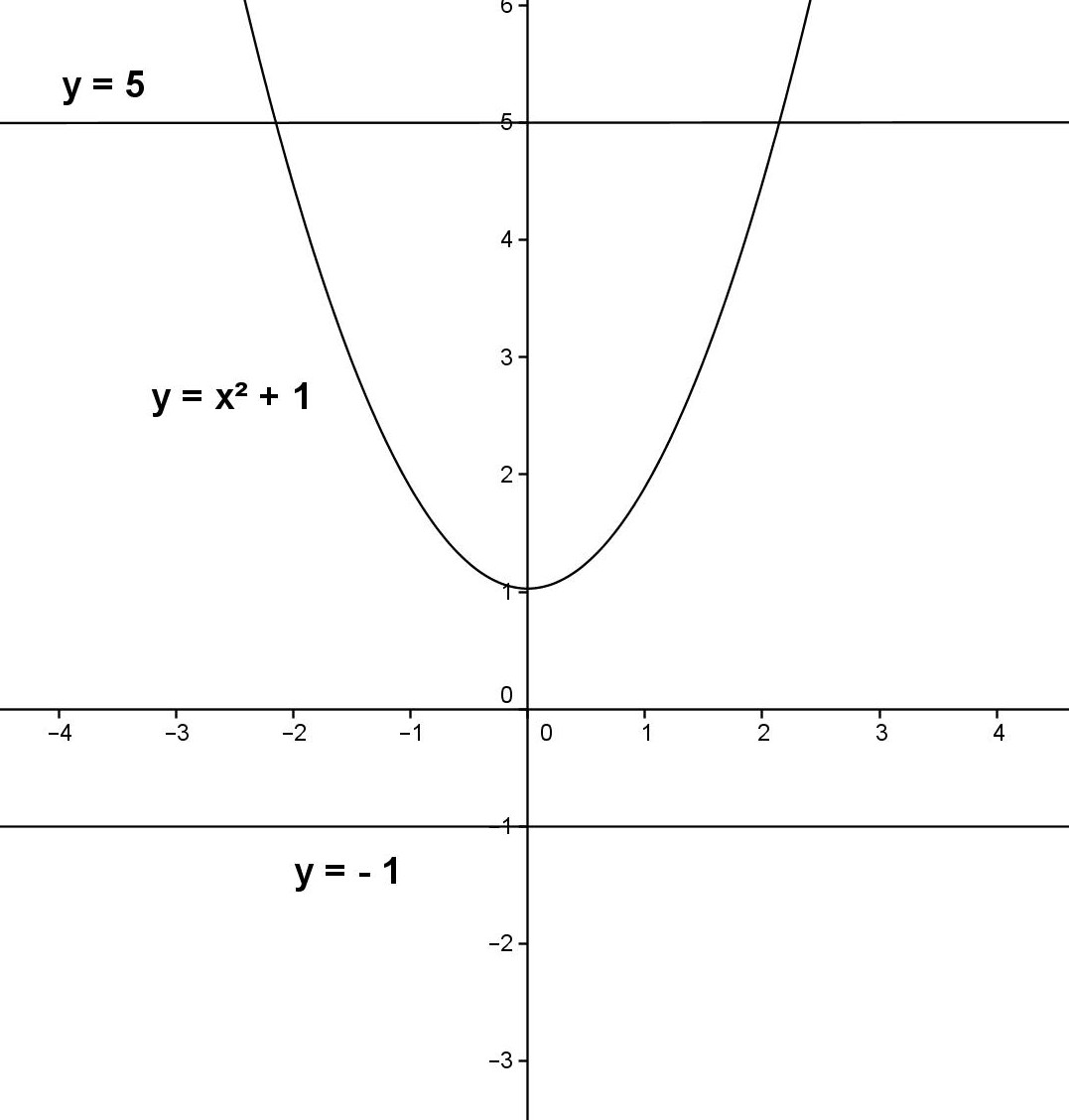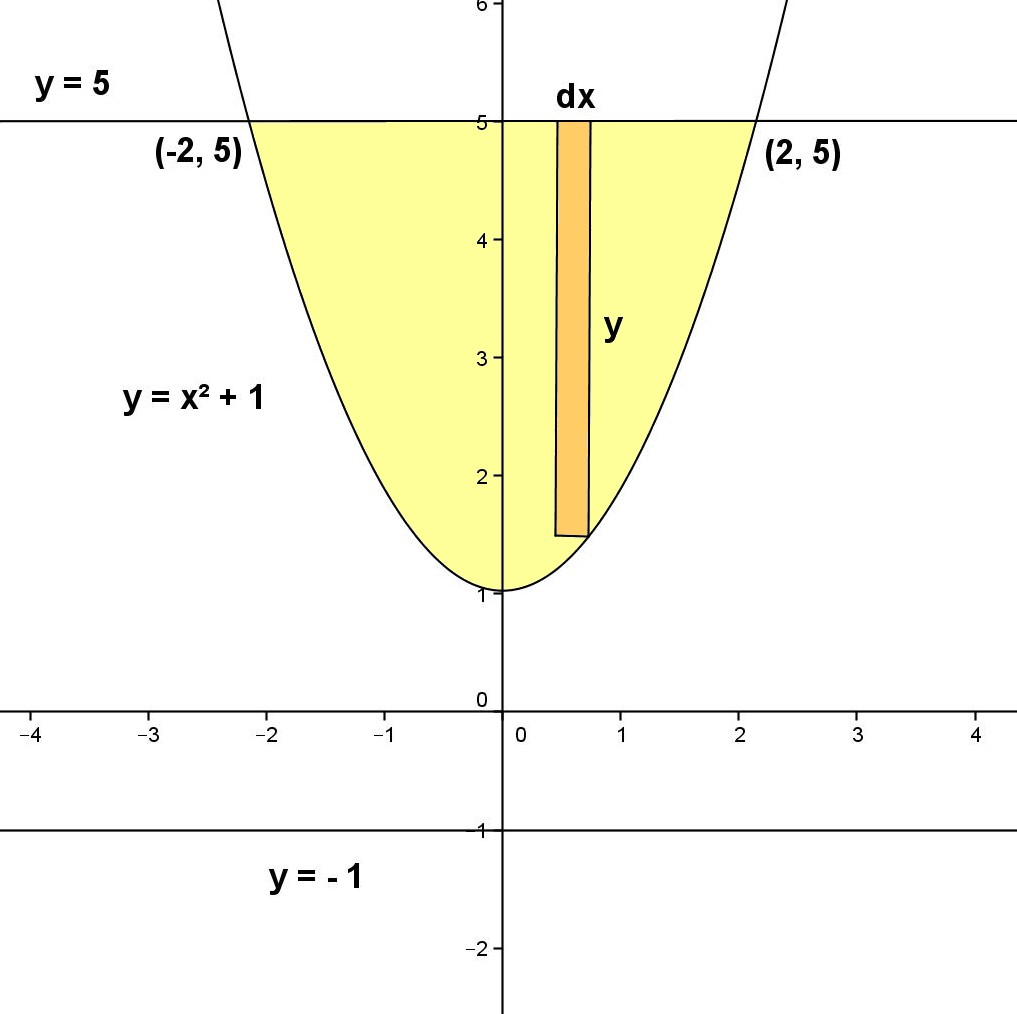## Friday, May 3, 2013

### Volume - Solid Revolution, 3

Category: Integral Calculus, Analytic Geometry, Algebra

"Published in Newark, California, USA"

Find the volume of the solid generated by revolving about y = -1 for the area bounded by the given curves.

Solution:

To illustrate the problem, it is better to sketch the graph of the two equations above using the principles of Analytic Geometry as followsPhoto by Math Principles in Everyday Life

From the figure above, there are two points of intersection between the two curves. Solve the systems of two equations two unknowns in order to get the coordinates of the points of intersection as follows

Equate
and

we have,

Therefore, their points of intersection are (-2, 5) and (2, 5).

Next, from the given two equations above, it is better to use a vertical strip at the area bounded by two curves and label further the figure as followsPhoto by Math Principles in Everyday Life

If you rotate the shaded area about y = -1, the vertical strip becomes a ring as followsPhoto by Math Principles in Everyday Life

The volume of a ring formed by the rotation of a vertical strip about the x-axis is

Integrate on both sides of the equation to get the volume of a solid formed by the rotation of the area about the x-axis as follows

Since the axis of rotation is NOT the x-axis as stated in the given problem, then we have to get the outer radius and inner radius first. The axis of rotation is one unit below the x-axis which is y = -1. The outer radius and inner radius of a disk will be increased by one unit as follows

Substitute the values of the variables to the above equation, we have

Simplify the fractions by getting their Least Common Denominator (LCD) and the volume of the solid of revolution along y = -1 is

## Thursday, May 2, 2013

### More Pyramid Problems

Category: Solid Geometry

"Published in Newark, California, USA"

What fraction of the volume of a pyramid must be cut off by a plane parallel to the base if the pyramid thus formed has a lateral area equal to one half of the lateral area of the original pyramid?

Solution:

To illustrate the problem, it is better to draw a pyramid with any base as followsPhoto by Math Principles in Everyday Life

If B1 is parallel to B2, then the two pyramids are similar. We can relate their lateral areas with their altitudes using the principles of ratio and proportion as follows

where S1 and S2 are the lateral area of the two pyramids, respectively. If S2 = 2S1, then the above equation becomes

Take the square root on both sides of the equation, we have

We can relate also their volumes with their altitudes using the principles of ratio and proportion as follows

but

Finally, their volume ratio is

## Wednesday, May 1, 2013

### Indeterminate Form - Zero Over Zero, 2

Category: Differential Calculus, Algebra

"Published in Newark, California, USA"

Evaluate

Solution:

Consider the given equation above

Substitute the value of x which is 0 to the above equation, we have

Since the answer is 0/0, then it is considered as Indeterminate Form which is not accepted as a final answer in Mathematics. Remember this, any number, except zero divided by zero is always equal to infinity. If the Indeterminate form is either 0/0 or /, then we can use the L'Hopital's Rule in order to solve for these type of Indeterminate Forms. In this case, we can apply the L'Hopital's Rule for the above equation as follows

Substitute the value of x which is 0 to the above equation, we have

Therefore,